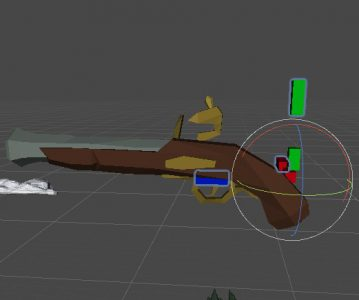# 如何反轉 Gyroscope (Quaternion) X-axis, Y-axis 輸入 ?

1. 在 Local Space 的 Quaternion 中把 X-axis 或 Y-axis 反轉的方法.
2. Gyroscope 是 Right hand 的輸入, 如何把 Right hand 轉換為 Left Hand
3. Gyroscope 的方向是 World space, 如何把 World space 轉換為遊戲中的 Local space.

## 反轉 Quaternion 某個軸的方法 (限 Local Space)

```private Quaternion GetPlayerInput(bool isFlipX, bool isFlipY)
{
Vector3 euler = GetBiasDeviceOrientation();
if (isFlipX) euler.x = -euler.x;
if (isFlipY) euler.y = -euler.y;
return Quaternion.Euler(euler);
}```

## Right hand 轉換為 Left hand

`private Quaternion GyroToUnity(Quaternion q) => new Quaternion(q.x, q.y, -q.z, -q.w);`

## World Space 轉為 Local Space

Low tech 的辦法是,

```Quaternion SampleRotation;
SampleRotation.Inverse() * SampleRotation; // This will zero out all rotation```

```Quaternion m_WorldSpaceOrientationBias;
private void Awake()
{
m_WorldSpaceOrientationBias = GyroToUnity(Input.gyro.attitude);
}

private Quaternion GetPlayerInput(bool isFlipX, bool isFlipY)
{
Vector3 euler = GetBiasDeviceOrientation();
if (isFlipX) euler.x = -euler.x;
if (isFlipY) euler.y = -euler.y;
return Quaternion.Euler(euler);
}
private Quaternion GetBiasDeviceOrientation()
{
return m_WorldSpaceOrientationBias.Inverse() * GyroToUnity(Input.gyro.attitude);
}
private Quaternion GyroToUnity(Quaternion q) => new Quaternion(q.x, q.y, -q.z, -q.w);```